Frank Mitchell

# Learning how a transmit filter works

The first practical circuit you build in The Electronics of Radio is a transmit filter. By practical, I mean the kind of circuit you could pull out of one design and drop into another. Something that fits in a black box. Here’s what the transmit filter in the NorCal 40A transceiver looks like.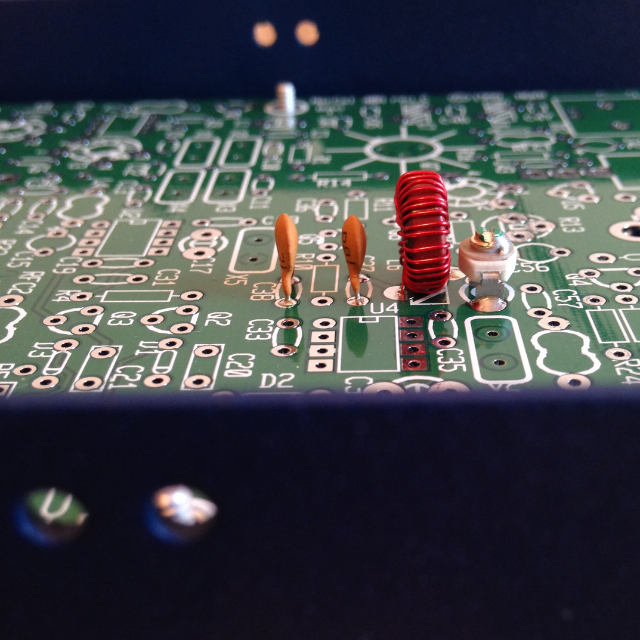From left to right those components are a 4.7 pF disc capacitor, a 100 pF disc capacitor, a hand wound 3.14 uH inductor, and a 50 pF variable capacitor. The schematic for them shows how they fit together electrically.## What’s a filter for?

What’s the point of a filter in a transmitter anyway? At its most basic, a transmitter consists of four parts. An antenna to radiate a signal, an oscillator to generate a signal, a power source to drive the oscillator, and a key to turn the power on and off.

The frequency the oscillator resonates at determines the frequency the transmitter broadcasts on. Most oscillators are made of quartz, which provides nice stable frequencies when you excite it with electrons. The problem with quartz is that it’s so stable, it doesn’t give you much tuning range. If your oscillator is a 7.114 MHz quartz crystal, you’re pretty much stuck transmitting on 7.114 MHz. Some radios, like the Rockmite II, are designed to be “rock bound” and operate on just one frequency.

The crystal oscillator in the NorCal 40A operates at 4.9150 MHz. This is known as the local oscillator or LO. Since 4.9 MHz isn’t in the 40 meter amateur radio band, we need to mix in a second signal to get things into the 7 MHz range. By making that second signal tunable, we can allow the radio to transmit over a range of frequencies.

That second signal comes from the variable frequency oscillator or VFO. The one in the NorCal 40A operates at 2.105 MHz. It’s got a bandwidth of about 40 kHz, which means you can tune it up or down by 20 kHz on either side. Mixing the LO’s 4.9 MHz signal with the VFO’s 2.1 MHz signal gives us the variable 7 MHz signal we want.

Signal mixing is known as heterodyning, and like most things that sound like they herald the dawn of Skynet, it has a down side. Not only does mixing signals get you their product, it gets you their difference as well. So we get the 7 MHz signal we want, but we also get a 2.8 MHz signal we don’t want. And that’s where the transmit filter comes in. Filters are used to remove unwanted signals.

## Making a minimal viable filter

You can make the worlds smallest filter by putting a capacitor and an inductor in parallel. This is known as an LC circuit, or a tuned circuit, and it stores electrical energy through oscillation.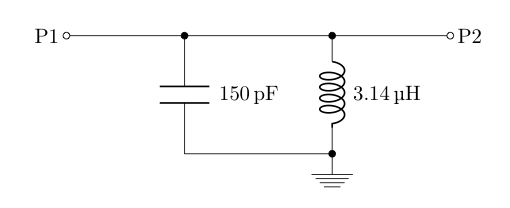A capacitor stores energy in an electric field. So if you connect a charged capacitor across an inductor, current will start to flow from the capacitor to the inductor and build up a magnetic field in the inductor. But the current doesn’t stop once the charge on the capacitor is gone. Because inductors resist changes in current, the capacitor will begin to charge back up, extracting energy from the magnetic field in the inductor. Back and forth it goes, from electric field to magnetic field, swapping polarity each time, and forming an oscillator.

You can calculate the frequency an LC circuit oscillates at if you know the inductance in henries and capacitance in farads of the components in it. Multiply the inductance by the capacitance and take the square root. Multiply that result by two pi and invert it.

ƒ = 1 / LC

The resulting frequency is in hertz, and it’s that tuned circuit’s resonant frequency. Because capacitors block low-frequency signals and inductors block high-frequency signals, a tuned circuit will only let signals at its resonate frequency through. Filters that behave like this are known as band-pass filters.

## Functions in filter land

The tuned circuit in the transmit filter of the NorCal 40A has a 100 pF capacitor, a 50 pF capacitor, and a 3.14 uH inductor. Because the two capacitors are in parallel, we can sum their values and pretend we’re working with a 150 pF capacitor. By plugging these values into the formula for resonate frequency, we can figure out where the circuit peaks.

7.33 MHz ≈ 1 / (3.14 × 10-6) ⋅ (150 × 10-12)

The peak of the filter lands just past the edge of the 40 meter band at 7.33 MHz. That’s good because it means the 7 MHz signal generated by the mixer will pass through the filter. But what about the 2.8 MHz signal we don’t want? How well does this filter remove that unwanted signal? To answer that, we have to look at the filter’s transmission loss.

Transmission loss is the ratio of the voltage that a circuit puts out to the voltage the circuit takes in. Here’s the function to calculate the transmission loss for an inductor and capacitor in parallel.

Vout2 / Vin2 = ω2L2 / R2(1 - ω2LC)2 + ω2L2

There are two variables in that function we don’t know. One is R, the input resistance of the filter in ohms. The other is ω (pronounced “omega”), the input frequency to the filter in radians per second. We can figure out the input resistance by looking at the circuit’s input. The transmit filter in the NorCal 40A is fed by a Philip’s SA602AN mixer. Digging through the data sheet for the mixer, we find that its output resistance is 1500 ohms. Which means the input resistance to our filter will also be 1500 ohms.

We know what the input frequency to our filter is, it’s 2.8 MHz. We can convert hertz to radians per second by multiplying by two pi.

17592919 rad/s ≈ 2π ⋅ 2800000

Now we know enough to figure out how good our filter is at rejecting a 2.8 MHz signal. We can take the formula for transmission loss and plug in 1500 ohms for R, 3.14 × 10-6 henries for L, 150 × 10-12 farads for C, and 17592919 radians per second for ω.

175929192 ⋅ 3.14e-62 / 15002 ⋅ (1 - 125663712 ⋅ 3.14e-6 ⋅ 150e-12)2 + 175929192 ⋅ 3.14e-62

Note that I’m being a little fast and loose with my notation here. Scientific e notation is usually reserved for calculators and programming languages, but I wanted to fit everything on one line.

Crunching through those numbers, we find our filter has a transmission loss of 0.0431 volts at 2.8 MHz. Transmission loss is usually expressed in decibels, which are a logarithmic scale where minus three decibels corresponds to a loss of half the voltage. We can convert volts to decibels by taking the base ten logarithm of the voltage and multiplying by twenty.

-27 dB ≈ 20 ⋅ log10(0.0431)

So our filter has a transmission loss of 27 dB at 2.8 MHz. In general, any frequency with a loss of 40 dB or more falls into what’s known as the stop band, the part where frequencies are rejected. Any frequency with a loss of zero to 3 dB is in the pass band, the part where frequencies are accepted. The range in between the pass band and the stop band is called the skirt.

Here’s a chart of our filter’s transmission loss for frequencies between 1 MHz and 15 MHz.

See the vertical line at 2.8 MHz and the horizontal line at -40 dB? We want our filter’s transmission loss curve to fall below that intersection point, because that will put the 2.8 MHz signal we don’t want in our filter’s stop band.

## Chaining filters together

To fix our filter, we need to add about 13 dB of cut off at 2.8 MHz to push it into the stop band. One way to get more loss out of filter is to stick several of them together in series. We know that capacitors block low-frequency signals, so we can stick a capacitor in series with our band-pass circuit and use that as an additional level of filtering.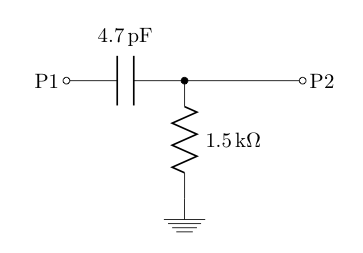The capacitor will block low-frequency signals and let high-frequency ones through. This kind of circuit is know as a RC circuit, or high-pass filter. We can find its peak frequency in hertz by multiplying its capacitance in farads by its resistance in ohms, multiplying that by two pi, and taking the inverse.

ƒ = 1 / 2π ⋅ RC

Since we know the input resistance to our band-pass filter is 1500 ohms, we’ll use that as the resistance in our high-pass filter. We can calculate the peak of our high-pass filter by plugging in 4.7 pF for C and 1500 ohms for R.

22.6 MHz = 1 / 2π ⋅ 1500 ⋅ 0.0000000000047

22.6 MHz is far away from the 7 MHz signal we care about, which is good, since it means we’ll get good loss at lower frequencies. But how much loss will we get? Here’s the formula for the transmission loss of a RC circuit.

Vout2 / Vin2 = ω2R2C2 / 1 + ω2R2C2

We already know that 2.8 MHz is 17592919 rad/s, so we can use that for ω. Plugging in 1500 for R and 3.14 pF for C, we can figure out how good our high-pass filter is at rejecting the unwanted signal.

0.1231 ≈ 175929192 ⋅ 15002 ⋅ 0.00000000000472 / 1 + 175929192 ⋅ 15002 ⋅ 0.00000000000472

Taking the base ten logarithm of the loss factor and multiplying by twenty gives us the loss in decibels.

-18 dB ≈ 10 ⋅ log10(0.1231)

Eighteen decibels of loss is plenty. If we graph the transmission loss equation, we can get a feel for how well it does across the rest of the band. The chart below goes from 1 MHz to 15 MHz.

That doesn’t look very promising for 7 MHz. If we crunch through the numbers, we find a loss of about 11 dB. Remember that three decibels of loss corresponds to a half loss of power. Because decibels is a logarithmic scale, you can convert decibels to a loss multiplier by dividing by ten and raising ten to the power of that result.

P = 10(dB / 10)

Interestingly, this formula explains why -40 dB was chosen as the cut off point for a filter’s stop band. It corresponds to a 10,000 times reduction in power. That’s like taking a 60 watt light bulb and reducing it to the 6 milliwatt smoldering ember on the end of a match stick.

Plugging in 11 for dB, we can figure out how much power we’re going to lose at 7 MHz with the addition of our high-pass filter.

13 ≈ 10(11 / 10)

Thirteen times! That seems like a lot. But there’s a side effect to having this capacitor in our filter. In addition to functioning as a high-pass filter, it also functions as a coupling capacitor. It’s going to remove any DC signals we might be getting from the mixer and only let AC signals pass through. That’s good, because our band-pass filter was designed with AC signals in mind.

The chart below shows what happens to our band-pass filter after we add the coupling capacitor to it. It looks very similar to the original band-pass filter plot, just with all the values shifted down. That’s actually another reason to work with transmission loss in decibels instead of volts. You can compute the total transmission loss of a series of chained filters by summing their individual losses in decibels.

Looking at the intersection of the vertical line at 2.8 MHz and the horizontal line at -40 dB, we can see that we’ve pushed our unwanted signal into the stop band. Yay!

There’s just one question left to answer. If we can do all this mathematical analysis, solving for losses at specific frequencies, why does the NorCal 40A include a 50 pF variable capacitor in its band-pass filter design? Why not just crunch the numbers and make it a fixed value?

## All the math is wrong!

It turns out that none of this math models reality at all. It’s all based on idealized circuits. When you buy a capacitor, even though it says 100 pF on it, it’s not actually 100 pF. There’s some tolerance level. It might be 100 pF plus or minus 10%. That means it’s really got a range of 90 pF to 110 pF.

There’s a point in Dave Richards’s build of the VK3YE Micro 40 DSB Transceiver where he says

Pin 1 of Peter’s LM386 is connected to ground via a 47uF cap and a 33 ohm resistor. I didn’t have a 47uF, but I did have a 33uF. Given the wide tolerances of electrolytics, it probably doesn’t matter much but I substituted a 33uF cap and a 47 ohm resistor.

When I first read that I was pretty shocked. What do you mean you dropped 14 uF of capacitance? This is electronics. It’s a practical application of physics. It’s science! You can’t just go swapping values in and out and hoping you arrive at something similar.

But as it turns out, you can. And you must. Because math is exact and the real world is not. For example, take a look at the inductor I wound for the transmit filter.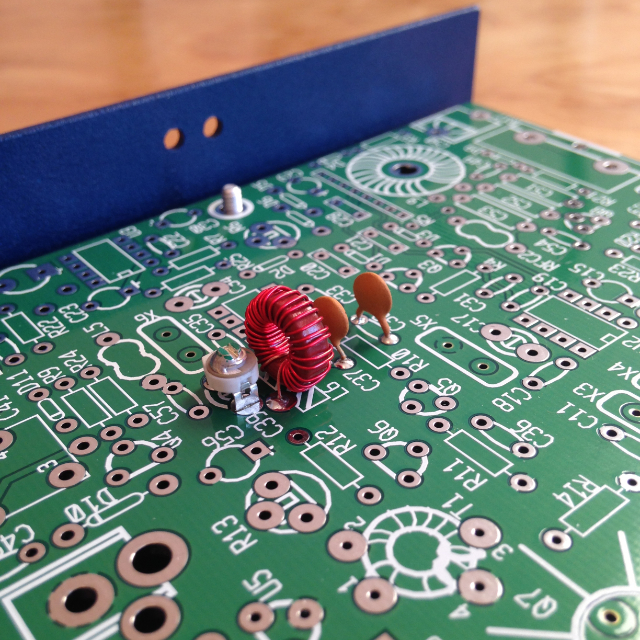That’s twenty-eight turns of No. 28 AWG magnet wire on a T37-2 core. Toroid cores have an constant that lets you compute their inductance based on the number of turns. Multiply the constant by the number of turns squared and divide the result by a thousand to get the inductance in micro henries.

L = AL ⋅ turns2 / 1000

The constant for a T37-2 core is 4 ± 5%. Plugging that in for AL and 28 for the number of turns, we can figure out the inductance.

3.14 uH ≈ 4 ⋅ 282 / 1000

But it’s not really 3.14 uH, because the math still assumes that the turns are spaced evenly and the wire lies flat against the core. Looking at the picture again, you can see that’s not the case. I used the chopstick trick by Jim Bullington, W6BUL, to wind that toroid, and I still ended up having to wind and rewind it several times before I was happy with the result.

The chart below is the band-pass filter for the NorCal 40A, but this time all the part tolerances have been taken into consideration. You can use the sliders below the chart to push the values around and watch the plot change.

There’s a reference plot in gray representing the behavior of the band-pass filter with exact ideal values. Notice that you can push C38 and L6 all the way to the left and there’s still enough swing in C39 to bring the filter’s behavior back in line with the ideal. Likewise if you push them all the way to the right, you’ve got enough variability to correct for that too.

So even though we can’t model reality exactly with math, we can model it well enough to get a feel for the behavior of a circuit. We can use it to predict new behavior relative to existing behavior when we add new components or substitute in new values. And we can use it get a deeper understanding of how a radio does what it does.

Finally, here’s one more shot of the assembled transmit filter. I really like how the light caught the bottom edge of the case and highlighted the bumpy metal texture.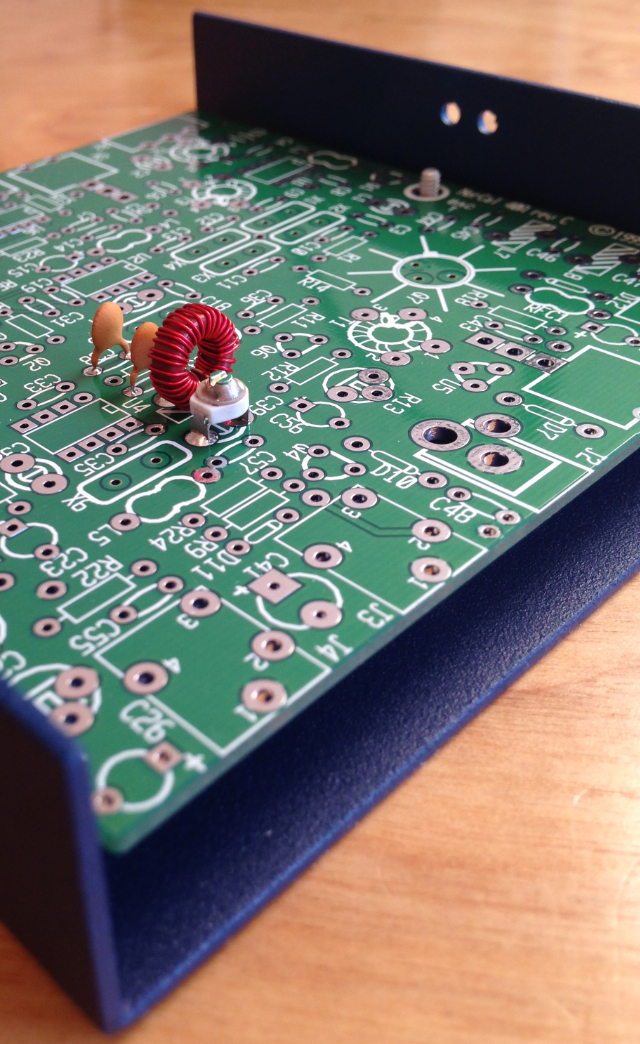## Where we go from here

This is the second part in a multi-part series about building the NorCal 40A transceiver. Links to the other parts are below.

1. Building my first HF radio
2. Learning how a transmit filter works
3. Regulating voltage with junk box parts

For the curious, photos where taken with an iPhone 5, cropped and resized with Acorn, and compressed with ImageOptim. Schematics where drawn with circuitikz and graphs where created with D3.